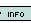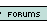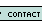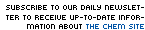1. Which statement is false: if a nonvolatile solute is added to a solvent, the vapor pressure of the solvent is lowered DHsolution">salts have a low DHsolution the amount of gas dissolved is directly proportional to the pressure of the gas above the solution heating always helps the solute dissolve in the solvent All statements are FALSE 2. A solution contains the same mass of two substances, substance A, which weights 200 g, and substance B, which weights 50 g. What is the mole fraction of substance A in this solution? 1/5 1/4 4/5 5/4 2/3 3. Van't Hoff's factor, i is incoporated into some equations due to a constant value that adjust for the pressure in the lab some electrolytes break up into ions a correction factor that makes data match up with hypothesis Van't Hoff factor I is a constant that corrects all osmotic equations none of the above 4. Which of the following would serve as the best electrical conductor (assuming constant molarities)? HC2H3O2 H2O H2CO3 H2SO4 HF 5. Calculate the pressure needed to prevent osmosis when 10.0 g KCl is added to 150 mL of water at 25oC. 20.1 atm 40.1 atm 65.7 atm 80.2 atm 160 atm Name: E-mail: Last 15 Challengersnews | about us | contact us tutorials index | organic chemistry | practice tests | online quizzes | reference tools site copyright (c) 2002 to neopages.com